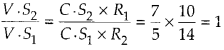Enlightened

# Question 10: NCERT Solutions for 12th Class Physics: Chapter 4-Moving Charges and Magnetism

• 0

Question 10: NCERT Solutions for 12th Class Physics: Chapter 4-Moving Charges and Magnetism

Two moving coil meters, M1 and M2 have following particulars: R1 = 10Ω, N1 = 30, A1 = 3.6 × 10-3 m2, B1 = 0.25 T R2 = 14 Ω, N2 = 42, A2 = 1.8 × 10-3 m2, B2 = 0.50 T. (The spring constants are identical for two meters). Determine ratio of (i) current sensitivity (ii) voltage sensitivity of M2 and A4,.

Share

1. Solution:
Current sensitivity of a moving coil galvanometer is defined as(ii) Ratio of voltage sensitivityCheck the complete chapter with solutions.

NCERT Solutions for 12th Class Physics: Chapter 4-Moving Charges and Magnetism

• 0